# 基于演化博弈论的电焊安全作业过程两方主体行为研究Research on Behavior of Two Parties in Welding Safety Operation Based on Evolutionary Game Theory

• 全文下载: PDF(475KB)    PP.317-323   DOI: 10.12677/MSE.2018.74038
• 下载量: 311  浏览量: 425

This paper studies and discusses the behavior decision-making of the two main bodies in the pro-cess of welding operation under the influence of each other, that is, whether the manager will strictly manage the welder’s operation behavior according to the regulations and whether the welder will carry out the welding operation according to the regulations. The formation, evolution path and influencing factors of behavior decision-making between two parties during welding op-eration are analyzed by constructing game model, in order to provide theoretical basis for avoiding operation risk of special types of work.

1. 引言

2. 模型假设与构建

$\left({a}_{1},{b}_{1}\right)=\left(-C,B\right)$ (1)

$\left({a}_{2},{b}_{2}\right)=\left(-C,\left(1+\alpha \right)B-\gamma P-E\right)$ (2)

$\left({a}_{3},{b}_{3}\right)=\left(0,B\right)$ (3)

$\left({a}_{4},{b}_{4}\right)=\left(-\theta T,\left(1+\alpha \right)B-\beta P\right)$ (4)Table 1. Income matrix of managers and welders during welding operation

3. 模型求解

3.1. 管理者的复制动态方程

${U}_{11}=y{a}_{1}+\left(1-y\right){a}_{2}=-C$ (5)

${U}_{12}=y{a}_{3}+\left(1-y\right){a}_{4}=-\left(1-y\right)\theta T$ (6)

${U}_{1}=x{U}_{11}+\left(1-x\right){U}_{12}$ (7)

$\frac{\text{d}x}{\text{d}t}=x\left({U}_{11}-{U}_{1}\right)=x\left(1-x\right)\left({U}_{11}-{U}_{12}\right)$ (8)

$F\left(x\right)=\frac{\text{d}x}{\text{d}t}=x\left(1-x\right)\left[\left(1-y\right)\theta T-C\right]$ (9)

3.2. 电焊工的复制动态方程

${U}_{21}=x{b}_{1}+\left(1-x\right){b}_{3}=B$ (10)

${U}_{22}=x{b}_{2}+\left(1-x\right){b}_{4}=\left(1+\alpha \right)B-\beta P-xE$ (11)

${U}_{2}=y{U}_{21}+\left(1-y\right){U}_{22}$ (12)

$F\left(y\right)=\frac{\text{d}y}{\text{d}t}=y\left(1-y\right)\left(\beta P+xE-\alpha B\right)$ (13)

4. 模型均衡分析

4.1. 管理者策略均衡分析

$y=1-\frac{C}{\theta T}$ 时， $F\left(x\right)\equiv 0$ ，这意味着对于所有的x取任何值该博弈都是稳定的。

$y\ne 1-\frac{C}{\theta T}$ 时，令 $F\left(x\right)=0$ ，可得到 $x=0$$x=1$ 两个稳定点。F(x)关于x求导可得 $\frac{\text{d}F\left(x\right)}{\text{d}x}=\left(1-2x\right)\left[\left(1-y\right)\theta T-C\right]$ ，此时可以分两种情况进行讨论。

1) 当 $0 时， ${\frac{\text{d}F\left(x\right)}{\text{d}x}|}_{x=0}>0$${\frac{\text{d}F\left(x\right)}{\text{d}x}|}_{x=1}<0$ 所以 $x=1$ 是平衡点，管理者选择严格管理策略是稳定的。

2) 当 $1-\frac{C}{\theta T} 时， ${\frac{\text{d}F\left(x\right)}{\text{d}x}|}_{x=0}<0$${\frac{\text{d}F\left(x\right)}{\text{d}x}|}_{x=1}>0$ ，所以 $x=0$ 是平衡点，管理者选择宽松管理策略是稳定的。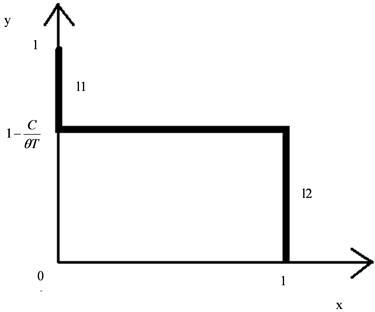Figure 1. Dynamic sketch of manager strategy evolution

4.2. 电焊工策略均衡分析

$x=\frac{\alpha B-\beta P}{E}$ 时， $F\left(y\right)\equiv 0$ ，这意味着对于所有的z取任何值该博弈都是稳定的。

$x\ne \frac{\alpha B-\beta P}{E}$ 时，令 $F\left(y\right)=0$ ，可得到 $y=0$$y=1$ 两个稳定点。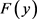关于y求导可得 $\frac{\text{d}F\left(y\right)}{\text{d}y}=\left(1-2y\right)\left(\beta P+xF-\alpha B\right)$ ，此时可以分两种情况进行讨论。

1) 当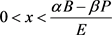时， ${\frac{\text{d}F\left(y\right)}{\text{d}y}|}_{y=0}<0$${\frac{\text{d}F\left(y\right)}{\text{d}y}|}_{y=1}>0$ ，所以 $y=0$ 是平衡点，电焊工选择违规作业策略是稳定的。

2) 当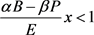时， ${\frac{\text{d}F\left(y\right)}{\text{d}y}|}_{y=1}<0$ ，所以 $y=1$ 是平衡点，电焊工选择规范作业策略是稳定的。

l3中，系统经过演化后电焊工的最终策略为违规作业，反之若初始状态位于直线中l4，则电焊工的最终策略为规范作业。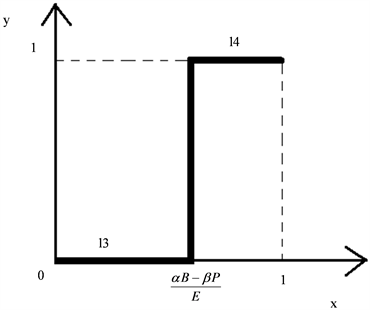Figure 2. Dynamic sketch of welder strategy evolutionTable 2. Evolutionary equilibrium state of two-party principal strategies in welding process

5. 两方演化博弈模型指导意义与安全管理对策

5.1. 两方演化博弈模型指导意义

5.2. 安全管理对策

1) 健全安全施工管理制度，完善奖惩机制。

2) 优化管理工程师素质，提高监督效率。

3) 优化施工团队素质，降低施工作业严格管理成本。

4) 加大宣传教育力度，增强风险意识。

  李豪峰, 高鹤. 我国煤矿生产安全监管的博弈分析[J]. 煤炭经济研究, 2004(7): 72-75.  付茂林, 黄定轩. 存在腐败的煤矿安全监察进化博弈分析[J]. 中国煤炭, 2006, 32(8): 67-69.  周文专. 基于系统动力学方法的安全库存水平控制研究[J]. 长沙民政职业技术学院学报, 2007, 14(1): 111-113.  董毓智. 煤矿安全管理存在问题面面观——兼及《职业健康安全管理体系》介绍[J]. 山西煤炭管理干部学院学报, 2007, 20(1): 86-87 + 93.  何刚, 张国枢, 陈清华 乔国通. 煤矿安全生产中人的行为影响因子系统动力学(SD)仿真分析[J]. 中国安全科学学报, 2008, 18(9): 43-47.